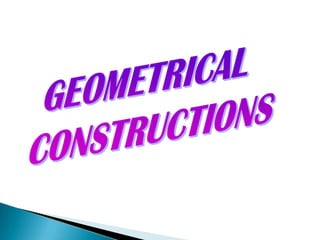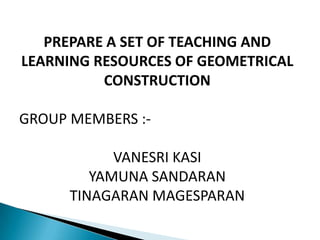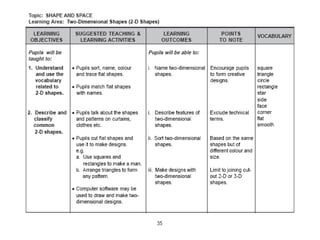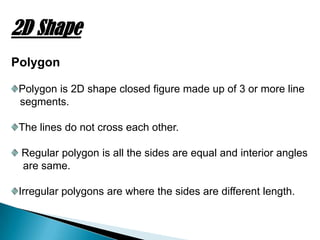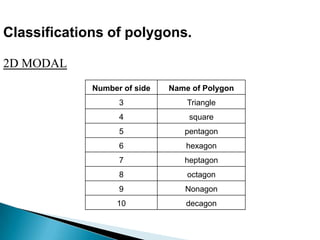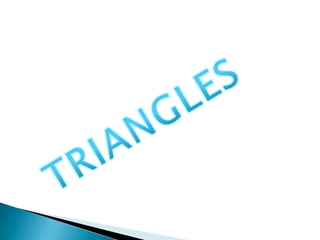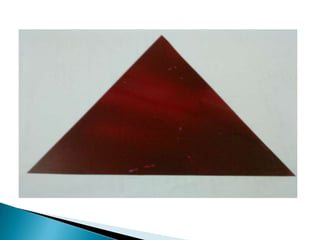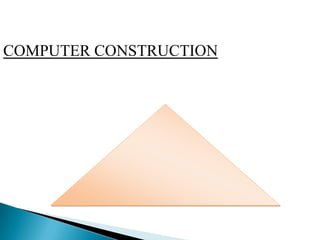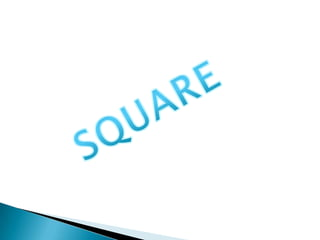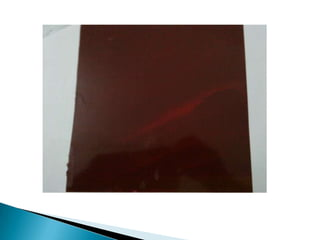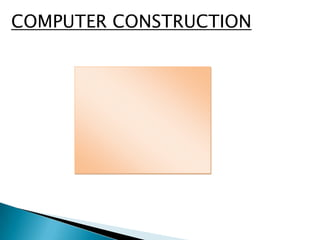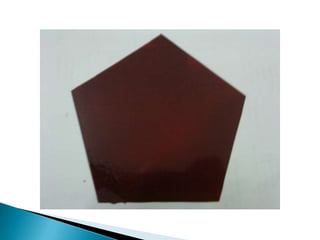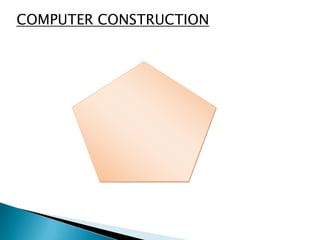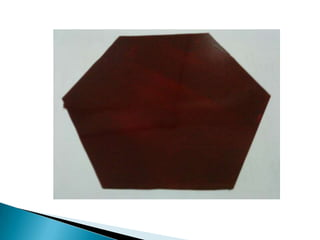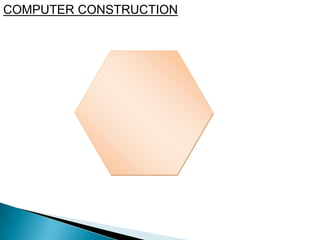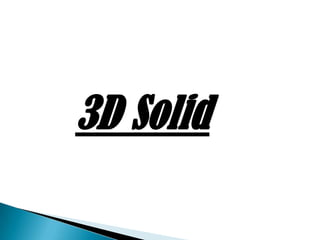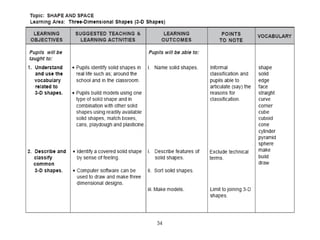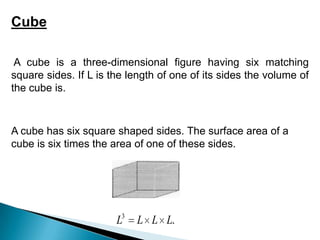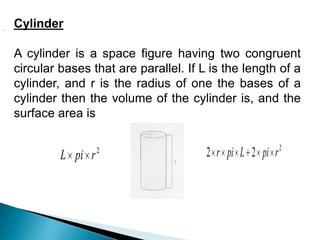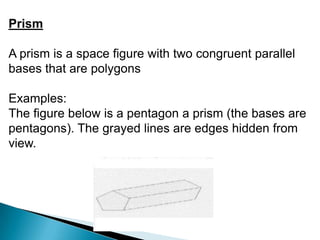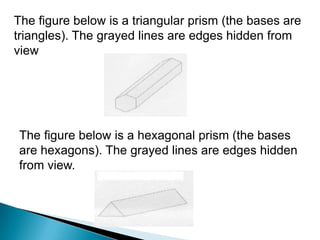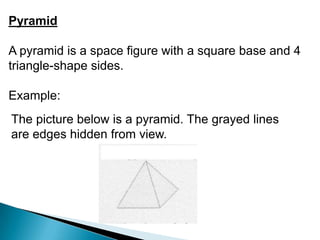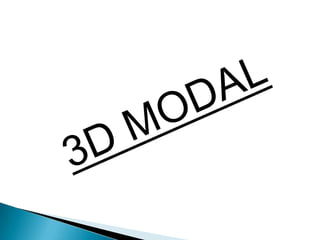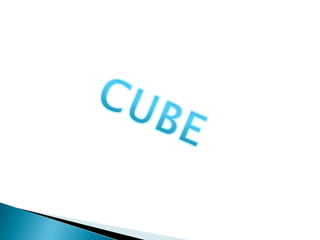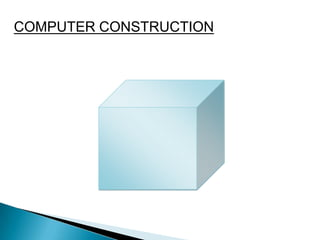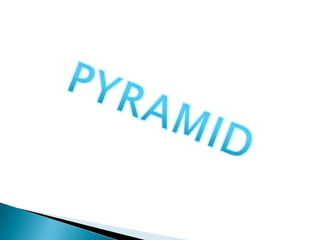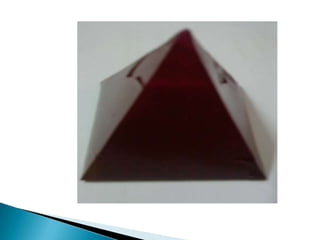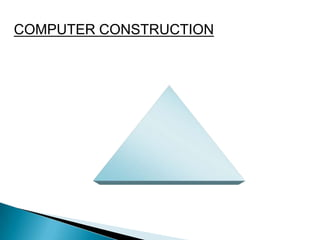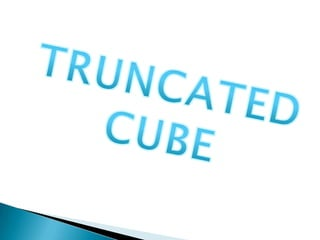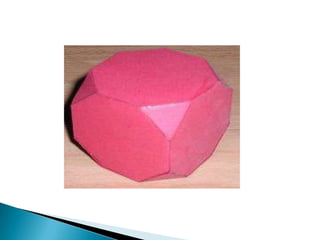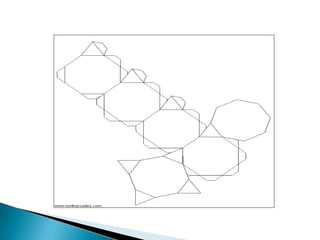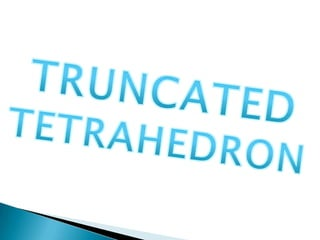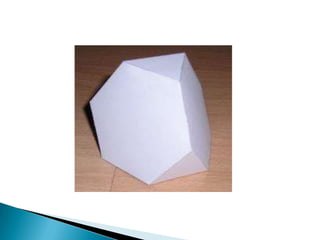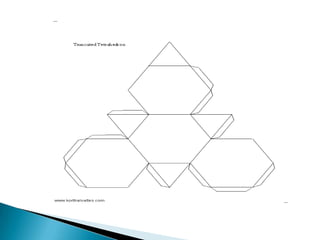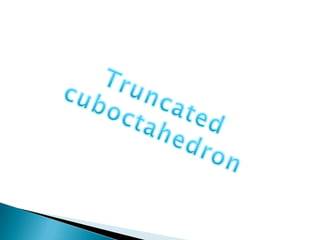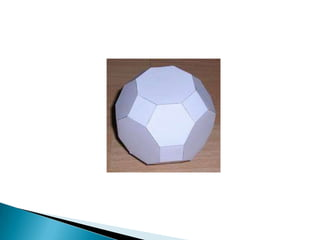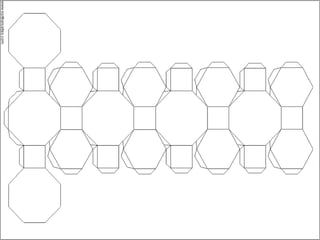1 of 40

### mathsproject-130321232400-phpapp02.pdf

1. PREPARE A SET OF TEACHING AND LEARNING RESOURCES OF GEOMETRICAL CONSTRUCTION GROUP MEMBERS :- VANESRI KASI YAMUNA SANDARAN TINAGARAN MAGESPARAN
2. 2D Shape Polygon Polygon is 2D shape closed figure made up of 3 or more line segments. The lines do not cross each other. Regular polygon is all the sides are equal and interior angles are same. Irregular polygons are where the sides are different length.
3. Number of side Name of Polygon 3 Triangle 4 square 5 pentagon 6 hexagon 7 heptagon 8 octagon 9 Nonagon 10 decagon Classifications of polygons. 2D MODAL
4. COMPUTER CONSTRUCTION
5. COMPUTER CONSTRUCTION
6. COMPUTER CONSTRUCTION
7. COMPUTER CONSTRUCTION
8. 3D Solid
9. . 3 L L L L Cube A cube is a three-dimensional figure having six matching square sides. If L is the length of one of its sides the volume of the cube is. A cube has six square shaped sides. The surface area of a cube is six times the area of one of these sides.
10. 2 r pi L 2 2 2 r pi L pi r Cylinder A cylinder is a space figure having two congruent circular bases that are parallel. If L is the length of a cylinder, and r is the radius of one the bases of a cylinder then the volume of the cylinder is, and the surface area is .
11. Prism A prism is a space figure with two congruent parallel bases that are polygons Examples: The figure below is a pentagon a prism (the bases are pentagons). The grayed lines are edges hidden from view.
12. The figure below is a triangular prism (the bases are triangles). The grayed lines are edges hidden from view The figure below is a hexagonal prism (the bases are hexagons). The grayed lines are edges hidden from view.
13. Pyramid A pyramid is a space figure with a square base and 4 triangle-shape sides. Example: The picture below is a pyramid. The grayed lines are edges hidden from view.
14. COMPUTER CONSTRUCTION
15. COMPUTER CONSTRUCTION